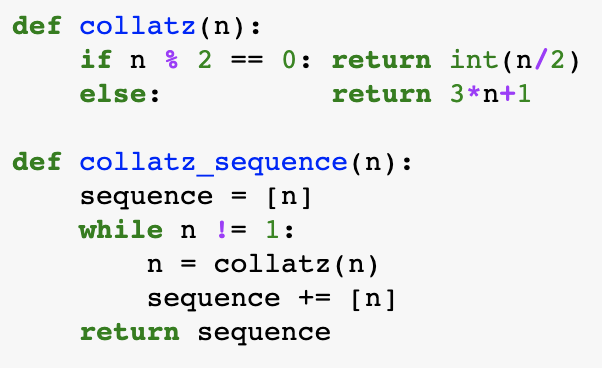The Collatz conjecture

Posted on Sun 02 June 2019 in Math • Tagged with python, math

The Collatz conjecture is a conjecture in mathematics that concerns a sequence defined as follows: start with any positive integer n. Then each term is obtained from the previous term as follows: if the previous term is even, the next term is one half the previous term. If the previous term is odd, the next term is 3 times the previous term plus 1. The conjecture is that no matter what value of n, the sequence will always reach 1.